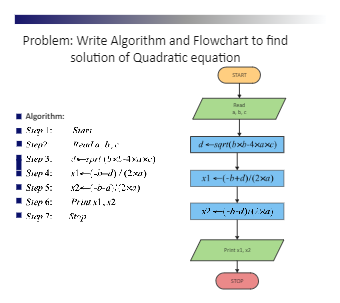Template Community / Quadratic Equation Algorithm FlowchartCharlotte
Published on 2021-07-16
Edit OnlineQuadratic equations are the polynomial equations of degree 2 in one variable of type: f(x) = ax^2 +bx + c, where a, b, c, ∈ R and a ≠ 0. The general form of the quadratic equation is called the leading coefficient, and c is called the absolute term of f(x). As shown in the diagram below, the nature of roots may be either real or imaginary. The general form of the quadratic equation is ax^2 + bx + c. The process starts by declaring the required variables and instructing the user to enter the coefficients of the quadratic equation by displaying suitable sentences using a print function. The System will initiate the scan function for the user to enter the point. Calculate the roots of quadratic equations using the proper formulae. Wait for the user to press a key using the get function to stop the function.
Tag
algorithm flowchart
Report
2
10.1kPostRecommended Templates